BayesMLE1

# Frequentist & Bayesian Statistics With Py4J & PyMC3¶

1. Introduction

2. Sampling A Distribution Written In Scala Using Py4J

3. The Maximum Likelihood Estimator

4. Confidence Intervals From Fisher Information

5. Bayesian Esimatators & Credible Intervals With PyMC3

6. Connecting The Two Methods

7. Conclusions

## 1. Introduction ¶

In this post I want to go back to the basics of statistics, but with an advanced spin on things. By "advanced spin" I mean, both from in terms of mathematics and computational techniques. The topic I'll dive into is:

Estimating a single parameter value from a distribution and then quantifying the uncertantity in the estimate.



In general I will take two approaches to quantitfying the uncertainity in the estimate, the first of which is frequentist and second that is Bayesian. I was originally inspired by Jake VanderPlas' post and admit that I am not very seasoned with Bayesian methods. That's why I'll be sticking to a simple example of estimating the mean rate or 𝜆 in a Poisson distribution from sampled data.

From the computational perspective, I wanted to do something different and decided to write the probability distribution for generating the data in Scala, but then use it with Python. Why did I do this? Well, I like Scala and enjoyed the challenge of writing a Poisson distribution using a functional approach. I also wanted to learn more about how to use Py4J which can be used to work with functions and objects in the JVM from Python. Apache Spark actually uses Py4J in PySpark to write Python wrappers for their Scala API. I've used both PySpark and Spark in Scala extensively in the past and doing this project gave me an opportunity to understand how PySpark works better.

The source code for this project can be found here.

Let's get into how I wrote the Poisson distribution in Scala and used it from Python to sample data.

## 2. Sampling A Distribution Written In Scala Using Py4J ¶

I wrote a Poisson distribution in Scala so that I could sample data from it to estimate the mean rate $\lambda$ of that distribution. The Poisson distribution is a probability distribution for a random variable $y \, \in \, \mathbb{Z}^{+}$ that represents some count phenomena, i.e. a number of non-negative integer occurences in some fixed time frame. For example the number of trains passing through a station per day or the number of customers that visit a website per hour can be modeled with Poisson distribution. The mathematical form of the distribution is,

$$p(y \, = \, k) \; = \; \frac{\lambda^{k} e^{-\lambda} }{k!}$$

The parameter $\lambda \, \in \, \mathbb{R}^{+}$ is the rate variable, i.e. the true number of customers that visit the website per hour and can be any non-negative real valued number.

The first step in this project was to create the Poisson class. I did this in a previous project, however, one key difference is for that Py4J the return value of any public function in Scala/Java needs to be a Java object. Specifically for me the sample method needs to return a Java List of integers (java.util.List[Int]). I originally tried returning a Scala List which worked fine in pure Scala, but when returning the list to Python I got a generic "Java Object" and realized Py4J was only able to serialize specific datatypes between Python and the JVM.

In order to use this class from Python with Py4J I needed to do three things:

1. Create a Gateway Server
2. Create a class entrypoint to allow for setting the Poisson attributes
3. Package the code as a jar using a build tool such as Maven or SBT

The first step is pretty straight forward to from the Py4J Documentation and is in the Main.Scala object. However, in order to accommodate the use of Docker I had to adapt the address for the GatewayServer based on this discussion on GitHub:

import java.net.InetAddress
import py4j.GatewayServer

object Main {
def main(args: Array[String]) = {
System.setProperty("java.net.preferIPv4Stack", "true");
val app = new PoissonEntryPoint()
val builder = new GatewayServer.GatewayServerBuilder(app)
val server = builder.build();
server.start()
println("Gateway Server Started")
}
}



The GatewayServer in the author's own words it allows Python programs to communicate with the JVM through a local network socket. The GatewayServer takes an entrypoint as a parameter which can be any object (see here for more info). However, the entrypoint doesn't really offer a way for us to pass the $\lambda$ value from Python to the Poisson constructor in Scala. To get around this issue I created a PoissonEntryPoint case class:

case class PoissonEntryPoint() {

def Poisson(lambda : Double) : PoissonDistribution = {
new PoissonDistribution(lambda)
}
}



This case class really just acts a Singleton, but is a class instead of an object. The point of the PoissonEntryPoint class is simply to be able to create a Poisson class with a specific $\lambda$ value after starting the GatewayServer.

Now let's talk about how the project is structured and how to package it for use. The project structure is:

src/
main/
scala/
Main.scala
PoissonDistribution.scala
PoissonEntryPoint.scala
pom.xml



The pom.xml file is called the project object model and is a file that contains all the instructions for Maven. I won't go into the details here, but I will say that Maven is a Java build tool to compile and package code and SBT is the Scala equivalent build tool. Since Scala is a JVM language we can use either build tool and I went with Maven since I'm more familiar with it and because it was much easier to find examples with Py4J using Maven than with SBT.

To package the code into a uber jar, use the command:

mvn package



Then we can start our our Py4J Web server with the command:

java -jar target/poisson-1.0-jar-with-dependencies.jar



We can test that the server is running on the default port 25333 on your local machine with the command,

nc -vz 0.0.0.0 25333



and you should see,

Connection to 0.0.0.0 port 25333 [tcp/*] succeeded!



Now we can start up our Jupyter notebook and connect Python to the JVM with the following code taken directly from Py4J's home page. This involves setting up the JavaGatway which is the main interaction point between a Python VM and a JVM. When running on your local machine this is simple, however, in order to use the my Poisson Distribution and Jupyter Lab within Docker Compose I had to pass the appropriate GatewayParameters which specify the address for the Scala GatewayServer (the py4jserver service in Docker compose) and the port it uses. In addition, I had to pass the CallbackServerParameters which specify the address for this notebook (the jupyter service in Docker compose) as well as the port it uses.

The callback server allows the JVM to call back Python objects as discussed here. I definitely had to have a friend that knows DevOps to help figure this one out, but it doesnt add too much complexity to the basic Py4J example:

In :
from py4j.java_gateway import JavaGateway, GatewayParameters, CallbackServerParameters

gateway = JavaGateway(
)

app = gateway.entry_point


The app is now the instantiated PoissonEntryPoint class. We can see the class type in Python

In :
type(app)

Out:
py4j.java_gateway.JavaObject

As well as looking at the methods for the class:

In :
dir(app)

Out:
['Poisson',
'apply',
'canEqual',
'copy',
'equals',
'getClass',
'hashCode',
'notify',
'notifyAll',
'productArity',
'productElement',
'productIterator',
'productPrefix',
'toString',
'unapply',
'wait']

We can see Poisson class method! Since PoissonEntryPoint is a case class it comes with a number of default methods just like a data class in Python.

We can then create a Poisson class instance and see that the value of $\lambda$ is 1.0:

In :
p1 = app.Poisson(1.0)


We can then instantiate another Poisson object:

In :
p2 = app.Poisson(3.0)


Note that the PoissonEntryPoint class has a function Poisson that returns a specific PoissonDistribution object that was initailized with the value $\lambda$. It is important that $\lambda$ is not an attribute of the PoissonEntryPoint othwerwise we would not get the seperate values of $\lambda$'s:

In :
p1.getLambda()

Out:
1.0
In :
p2.getLambda()

Out:
3.0

The really nice thing about Py4J is that you can treat objects in the JVM as if they are Python objects. For instance we can see the methods in the object:

In :
dir(p1)

Out:
['$anonfun$cdf$1', '$anonfun$getSum$1',
'$anonfun$invCDF$1', '$anonfun$invCDF$2',
'$anonfun$invCDF$3', '$anonfun$invCDF$4',
'$anonfun$sample$1', '$anonfun$uniform$1',
'$lessinit$greater$default$1',
'cdf',
'equals',
'getClass',
'getLambda',
'getSum',
'hashCode',
'invCDF',
'notify',
'notifyAll',
'prob',
'sample',
'setLambda',
'toString',
'uniform',
'wait']

We can then just use the methods in the PoissonDistribution object just like they would be used directly in Scala. For instance we can get the probability of $y=1$ when $\lambda = 1$:

In :
p1.prob(1)

Out:
0.36787944117144233

Now let's generate a random samle from the Poisson object:

In :
sample = p1.sample(1000)

In :
sample[:3]

Out:
[2, 1, 1]

It looks like Py4J returns a Python list while the PoissonDistribution class returns a java.util.List[Int]:

In :
type(sample)

Out:
py4j.java_collections.JavaList

We can then convert it to a Python list

In :
type(list(sample))

Out:
list

As mentioned previously, Py4J can only serialize specific Java objects back to Python, but I think that's still awesome! This is also why I needed to convert to from a Scala List[Int] to a java.util.List[Int]; without it the returned object would just be a generic Java Object and I wouldnt be able to access its contents.

Now let's visualize the Poission distribution for different values of $\lambda$

In :
import pandas as pd
import seaborn as sns

df = pd.melt(
pd.DataFrame({
'1':list(app.Poisson(1.0).sample(100)),
'2':list(app.Poisson(2.0).sample(100)),
'3':list(app.Poisson(3.0).sample(100)),
'4':list(app.Poisson(4.0).sample(100)),
'5':list(app.Poisson(5.0).sample(100)),
'6':list(app.Poisson(6.0).sample(100))
}),
var_name=["lambda"]
)

sns.displot(df, x="value", hue="lambda", kind='kde', height=5)

Out:
<seaborn.axisgrid.FacetGrid at 0x7fb35e47ef90>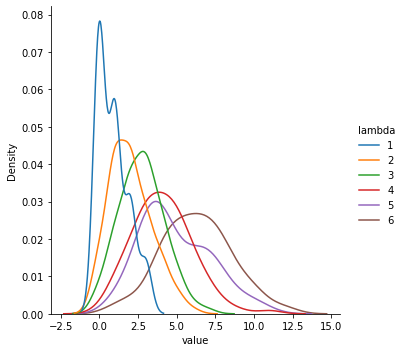Note that the negative values are not real, but an artifact caused by interpolation with Kernel Density Esimation. The same is true with the wiggles in the distribution.

We can verify this the former,

In :
df.query("value < 0")

Out:
lambda value

Now let's get into the Maximum Likelihood Estimator for $\lambda$ using the distribution p1.

## 3. The Maximum Likelihood Estimator ¶

First what is the difference between a statistic and an estimator? A statistic is any function of a sample. An estimator is any function of a sample that is used to estimate a population parameter. The maximum likelihood estimator is the value of a population distribution $\lambda$ that maximizes the probability of observing the sample.

We can find the MLE from a independent, identically distributed sample $y_1, y_2, \, \ldots \,, y_{n}$ from $f(y \, \vert \, \lambda)$ by defining the likelihood function,

$$L(\lambda \, \vert \, y_1, \, y_2, \, \ldots, \, y_n) \; = \; \prod_{i=1}^{n}f(y_{i} \, \vert \, \lambda)$$

Then we can find the MLE $\widehat{\lambda}$ such that,

$$\hat{\lambda}_{n} \; = \; \max_{\lambda} \, L(\lambda \, \vert \, y_1, y_2, \ldots, \, y_n)$$

From calculus we know that we can find the maximum (or minimum) of any function by solving,

$$\frac{\partial L(\lambda \, \vert y_1, y_2, \ldots, y_n)}{\partial \lambda} \; = \; 0$$

for $\lambda$. The MLE has many important properties, the most important in my mind are some are,

1. It is a consistent estimator.
2. It is invariant, so that if $\widehat{\lambda}$ is the MLE for $\lambda$, then for any function $\tau(\lambda)$, the MLE for $\tau(\lambda)$ is $\tau(\widehat{\lambda})$.
3. The MLE is an asymptotically normal estimator. That is $\widehat{\lambda} \; \sim \; N(\lambda, \, \mathcal{I}^{-1})$.

To explain the first property we, must note that since an estimator is a function of the sample space, it is also a random variable. Let $X_1, \, \ldots, \, X_n$ be a sequence of random variables then $X_{i} \; \xrightarrow{\mathcal{P}} \; X$ if,

$$\forall \epsilon > 0, \; \lim\limits_{n \rightarrow \infty} P(\vert X_i - X \vert > \epsilon ) \; = \; 0$$

then we say the random variable converges in probability. For an estimator this property of convergence is called consistency. Consistency is a necessary condition of any estimator in statistics and basically signifies that estimator eventually settles down to constant or some distribution of values.

The second property of the MLE allows us to transform our likelihood function into one that is often easier to calculate the MLE with, i.e. the log-likelihood function. That is the MLE, $\hat{\lambda}$ will satisfy,

$$\frac{\partial \log(L(\lambda \, \vert y_1, \, \ldots, y_n ))}{\partial \lambda} \; = \; 0$$

The third property of the MLE, of asymptotic normality, is helpful in modeling since your standardized residuals are normal. Hence the sum of squares of the residuals are $\chi^2$ distributed. This allows us to define confidence intervals around of estimates. The term $\mathcal{I}$ is the Fisher Information and will be discussed in the next section.

For the Poisson distribution the likelihood function is,

\begin{align} L(\lambda \, \vert y_1, \, y_2, \, \ldots, \, y_n) \; &= \; \prod_{i=1}^{n} \frac{e^{-\lambda} \, \lambda^{y_i}} {y_i!} \newline &= \; e^{-n \, \lambda} \lambda^{n \, \bar{y}_{n}} \frac{1} {\prod_{i=1}^{n} y_i!} \end{align}

We take the log of both sides and then setting the derivative equal to zero we find

$$- n \, + \, \frac{n \, \bar{y}_{n}}{\widehat{\lambda}} \, = \, 0$$

Then solving for $\hat{\lambda}$ we find the MLE is,

$$\widehat{\lambda} \, = \, \bar{y}_{n}$$
In :
from typing import List

def mle(sample: List[int]) -> float:
converted = list(sample)
return sum(converted) / len(converted)

lam = mle(p2.sample(1000))

print(f"lambda = {lam}")

lambda = 2.97


Our estimate for $\lambda$ is pretty close to the true value of 3 which is correct for p2!

Now, since the maximum likelihood estimator is the mean we know it satifies the Central Limit Theorem,

$$\hat{\lambda} _{n}\, = \, \bar{y}_{n} \; \xrightarrow{\mathcal{D}} \; N(\lambda,\lambda/n)$$

Hence we can repeatedly sample p2 and compute the distribution of the MLE for various values of sample size $n$ to show how the MLE converges in distribution.

In :
import numpy as np

In :
# sample the MLE 100 times for each n = 10, 50, 100, 500, 1000
samples = [ [ mle(p2.sample(n)) for k in range(100)] for n in [10, 20, 50, 100, 200, 500, 1000]]

In :
sample_df = pd.melt(
pd.DataFrame(np.array(samples).T,
columns=['10', '20', '50','100', '200', '500', '1000']),
var_name=["n"]
)

# # plot the MLE for various value of sample size
sns.displot(sample_df, x="value", hue="n", kind="kde", height=5,)

Out:
<seaborn.axisgrid.FacetGrid at 0x7ff00dba4090>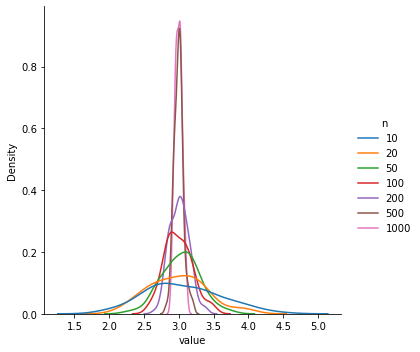As $n \rightarrow \infty$ we see the MLE $\bar{y}_{n}$ has a distribution that is more sharply peaked around $3$ and hence shows that the esimator is converging to the true value!

We have seen that the MLE $\hat{\lambda}_{n}$ converges to the true value of $\lambda$, but for any finite value of $n$ the esimator can be incorrect. How do we measure our confidence in our estimae $\hat{\lambda}_{n}$? The answer is using confidence intervals.

## 4. Confidence Intervals From Fisher Information ¶

Given a distribution $y_{i} \, \sim \, f(y \, \vert \, \alpha)$ for $i \, = \, 1, \ldots , n$ and the likelihood function,

$$L(\alpha \, \vert \, y_1, y_2, \ldots, y_n) \; = \; \prod_{i=1}^{n} \, f(y_i \, \vert \, \alpha)$$

We define the score statistic) $S(\alpha)$ to be,

$$S(\alpha) \; = \; \frac{d\log \left(L(\alpha \, \vert \, y_1, y_2, \ldots, y_n) \right)}{d\alpha}$$

For a distribution from the exponential family the score function satisfies,

$$E_{y}[S] \; = \; 0$$

Another important property of the score statistic is that it satisfies,

$$S(\widehat{\alpha}) \; = \; 0$$

for the MLE $\widehat{\alpha}$. This property can be used to compute the MLE using the so-called scoring algorithm which is equivalent to Newton–Raphson method. The later method is more frequently used in calculations as it is a general optimization method and has many efficient implmentations.

The Fisher information is defined as,

\begin{align} \mathcal{I} \; &= \; \text{Cov}[S] \\ & = \; \text{Var}[ S S^{T}] \\ & = \; E_y[ - S'] \\ &= \; - E_{y} \left[ \frac{d^{2}\log \left(L(\alpha \, | \, y_1, y_2, \ldots, y_n) \right)}{d\alpha^{2}} \right] \end{align}

One can show that the standard error for the maximum likelihood estimate $(\widehat{\alpha})$ will then be,

$$\text{S.E.}(\widehat{\alpha}) \; = \; \mathcal{I}^{-1/2}$$

The curvature of the log-likelihood at the MLE is dictated by the Fisher information. If $L$ flat at the MLE then the $\mathcal{I}$ is small and the MLE is not stable or well-defined. Higher Fisher information at the MLE means the distribution is highly peaked and implies the MLE is well defined and stable.

As previously mentioned the MLE asymptotically normal which tells us mathematically that,

\begin{equation} \widehat{\alpha} \; \sim \; N(\alpha, \, \mathcal{I}^{-1}) \end{equation}

These facts can be used to calculate confidence intervals for the MLE,

$$\text{CI}_{\alpha} \, = \, [ \widehat{\alpha} - Z_{\alpha/2} \, \mathcal{I}^{-1}/\sqrt{n}, \, \widehat{\alpha} + Z_{\alpha/2} \, \mathcal{I}^{-1} /\sqrt{n}]$$

The Fisher information for a Poisson distribution is,

$$\mathcal{I} \, = \, 1/\lambda$$

This means for our MLE of the Poisson distribution the confidence interval will be:

$$\text{CI}_{\hat{\lambda}} \, = \, [ \bar{y}_{n} - Z_{\alpha/2} \, \sqrt{\bar{y}_{n} / n}, \, \bar{y}_{n} + Z_{\alpha/2} \, \sqrt{\bar{y}_{n}/ n}]$$

We can then come up with a functin to compute the 94% confidence interval (most people choose 95%, but to be consistent with PyMC3 we use 94%) for the sample:

In :
from typing import Tuple

def ci(sample: List[int]) -> Tuple[float,float]:
"""
Computes the 94% confidence interval for sampled
data from a Poisson distribution
"""
z = 1.88
m = mle(sample)
n = len(sample)
return (m - z*np.sqrt(m/n), m + z*np.sqrt(m/n))


We can then get the MLE for the sampled data from Poisson(1.0),

In :
mle(sample)

Out:
0.995

The 94% confidence interval is then,

In :
ci(sample)

Out:
(0.9356979932885909, 1.054302006711409)

We can see that the confidence interval does contain the true $\lambda \, = \, 1$. Many people think a 94% confidence interval, $\text{CI}_{94}$, can be used to say that there is a 94% probability that the true $\lambda$ is in the confidence interval $\text{CI}_{94}$. This interpetation is wrong, in frequentist methods, the parameter $\lambda$ is assumed to be an unknown fixed value. One cannot make probability statements about fixed values.

The confidence interval is a function of the sample space and therefore a random variable. One can make probability statements about the confidence intervals. Indeed the correct interpretation is that if you are able to repeatedly re-sample the population distribution $f(y \, \vert \, \lambda)$ to form many confidence intervals $\text{CI}_{94}$, 94% of them would contain the true population parameter $\lambda$.

We can test this by creating a function which returns a boolean indicating whether or not the parameter lam for $\lambda$ is contained in the 94% confidence interval from the data sample:

In :
def in_ci(lam: float, sample:  List[int]) -> bool:
interval = ci(sample)
return (lam >= interval and lam <= interval)


We can then test this function,

In :
in_ci(1, sample)

Out:
True
In :
in_ci(3, sample)

Out:
False

We can loop over 1,000 confidence intervals to see how many times they capture the true rate parameter,

In :
count_in_ci = [1 if in_ci(1, p1.sample(1000)) else 0 for i in range(1000)]
print("Confidence interval captures true rate {}% of times".format(100*sum(count_in_ci)/len(count_in_ci)))

Confidence interval captures true rate 94.3% of times


This is nearly spot on to what the theory says! Let's now move on to Bayesian methods!

## 5. Bayesian Esimatators & Credible Intervals With PyMC3 ¶

In the frequentist approach the parameter we wish to estimation $\lambda$ is fixed, but unknown. The observed data $\left\{ y_1, y_2, \ldots, y_n \right\}$ is assumed to be from a population $f(y \, \vert \, \lambda)$ and estimates about the value of the population paremeter $\lambda$ is obtained by using the maximum likelihood. As we discussed above, probability statements about the unknown rate constant $\lambda$ don't make sense as its a fixed value and not a random variable. However, probability statements can be made about a confidence interval for $\lambda$. In the maximum likelihood method, asympotic normality allows us to use confidence intervals as a way to quantify the uncertaintity in our estimator.

In contrast, in Bayesian statistics, $\lambda$ is not a fixed value, but assumed to have values coming from a probability distribution called the prior $P(\lambda)$. This is often subjective and the choice of distribution for the prior often comes from domain knowledge. The observed data $\left\{ y_1, y_2, \ldots, y_n \right\}$ and samples from the prior taken to evaluate the likelihood of the posterior distribution model, $P(\lambda \, \vert \, y_1, y_2, \ldots, y_n )$. Now we can formulate estimators for $\lambda$ and quanitify the uncertaintity in those esimates by directly using the posterior distribution.

Let $P(y_1, y_2, \ldots, y_n \, \vert \, \lambda )$ be the sampling distribution then Baye's theorem states,

$$P(\lambda \, \vert \, y_1, y_2, \ldots, y_n) \; = \; \frac{ P ( y_1, y_2, \ldots, y_n \, \vert \, \lambda) \, P(\lambda)}{m(y_1, y_2, \ldots, y_n)}, \quad \text{and} \qquad m(y_1, y_2, \ldots, y_n) \; = \; \int P ( y_1, y_2, \ldots, y_n\, \vert \, \lambda) \, P(\lambda) \, d\lambda$$

Where $m(y_1, y_2, \ldots, y_n)$ is called the marginal distribution and used for normalization. Another way to rewrite Baye's formula is in terms of the Likelihood functions, $L(\lambda \, \vert \, y_1, y_2, \ldots, y_n)$

$$P(\lambda \, \vert \, y_1, y_2, \ldots, y_n) \; = \; \frac{ L(\lambda \, \vert \, y_1, y_2, \ldots, y_n) \, P(\lambda)}{m(y_1, y_2, \ldots, y_n)}$$

The Bayesian estimator (often called the posterior mean) is taken the be the expected value over the the random variable $y$,

$$\widehat{\lambda} \; = \; E_{\lambda \, \vert \, y}(\lambda \, \vert \, y_1, y_2, \ldots, y_n)$$

Until the advant of computers, statisticians were stuck with using conjugate priors with Bayesian methods since there are analytic solutions for the posterior distribution.

The conjugate prior for a Poisson distribution is a Gamma distributed which for $\alpha, \beta > 0$ takes the form,

$$P(\lambda \, \vert \, \alpha, \beta ) \; = \; \frac{\beta^{\alpha }}{(\alpha-1)! } \, x^{\alpha-1} \, e^{-\beta y}$$

A depiction of the Gamma distribution for various values of $\alpha$ and $\beta$ can be seen from PyMC3's website.Then the posterior is again a Gamma distribution, but with $\alpha^{\prime} \, = \, \alpha \, + \, n$ and $\beta^{\prime} \, = \, \bar{y}_{n}, \, \beta \, + \, n$. This leads to a posterior mean,

$$\hat{\lambda} \; = \; \frac{\alpha}{\beta \, + \, n} \, + \, \frac{ \bar{y}_{n}}{1 \, + \, \beta \, / \, n\, }$$

We can see that with little data (small $n$) our estimate we are closer to the prior mean ($\frac{\alpha}{\beta}$) while with lots of data (large $n$) we move towards the average $\bar{y}_{n}$.

Let's see this convergence for ourselves! We can define the posterior mean function:

In :
def posterior_mean(alpha: float, beta: float , sample: List[int]) -> float:
n = len(sample)
m = sum(sample) / n

return alpha / (beta + n) + m  / (1 + beta / n)


Then define a 1,000 random samples of various sizes:

In :
from random import randint
nums_samples = [randint(2,1000) for i in range(1000)]
nums_samples.sort()


We can the calculate and plot the posterior mean for the Poisson-Gamma model using the data and $\alpha \, = \, 1$ and $\beta \, = \, 3$,

In :
alpha = 3.0
beta = 1.0

In :
samples = [ posterior_mean(alpha=alpha, beta=beta, sample = p1.sample(n))
for n in nums_samples]

(pd.DataFrame({
"posterior_mean":samples,
"sample_size":nums_samples
}).plot(x="sample_size",
y="posterior_mean",
title="Covergence of Posterior Mean",
ylim=(0,1.75)))

Out:
<AxesSubplot:title={'center':'Covergence of Posterior Mean'}, xlabel='sample_size'>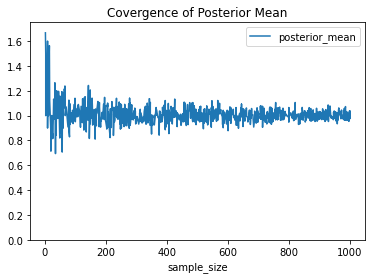Now let's talk about the confidence of this estimate. The anology of confidence in Bayesian esimation is called the credible Interval which requires the full posterior. I wrote a function to plot the posterior for the sample from p1 below,

In :
from gamma import posterior_distribution

posterior_distribution(alpha, beta, sample)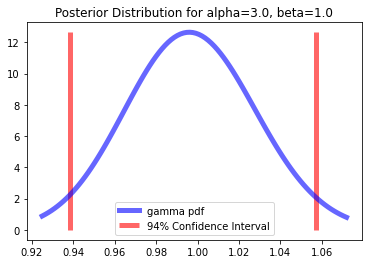As we saw the Bayesian estimator requires the full posterior distribution. Without a conjugate prior Bayesian methods requires numerical approximation to the posterior which computationally expenisve. Despite the added complexity, Bayesian methods allow us to handle situations where we might not have much data and can often lead us to estimates with smaller variance.

One approach to approximating the posterior distribution is to randomly sample the the prior distribution and then evaluate the likelihood of that prior value and the data using Bayes rule,

$$P(\lambda \, \vert \, y_1, y_2, \ldots, y_n) \; = \; \frac{ L(\lambda \, \vert \, y_1, y_2, \ldots, y_n) \, P(\lambda)}{m(y_1, y_2, \ldots, y_n)}$$

Repeatedly sampling the prior and evaluating the likelihood multiple times gives us a good approximation to the posterior distribution. Once we have the posterior distribution we can then evaluate the expected value of $\lambda$. A common method for generating the random samples of the prior above is through Markov Chain Monte Carlo Methods. Bayesian methods with MCMC can be used easily with PyMC!

We begin by importing the library

In :
import pymc3 as pm


Then define the model as the same Poisson-Gamma above, and sample it 5,000 times to get the expected mean:

In :
with pm.Model() as model_1:
λ = pm.Gamma('λ', alpha=3, beta=1)
y = pm.Poisson('y', mu=λ, observed=list(sample))
trace = pm.sample(5000, tune=2000, return_inferencedata=True)

Auto-assigning NUTS sampler...
Sequential sampling (2 chains in 1 job)
NUTS: [λ]

100.00% [7000/7000 00:14<00:00 Sampling chain 0, 0 divergences]
100.00% [7000/7000 00:14<00:00 Sampling chain 1, 0 divergences]
Sampling 2 chains for 2_000 tune and 5_000 draw iterations (4_000 + 10_000 draws total) took 29 seconds.


We can then view the posterior distribution using the ArviZ library,

In :
import arviz as az
az.plot_posterior(trace)

Out:
<AxesSubplot:title={'center':'λ'}>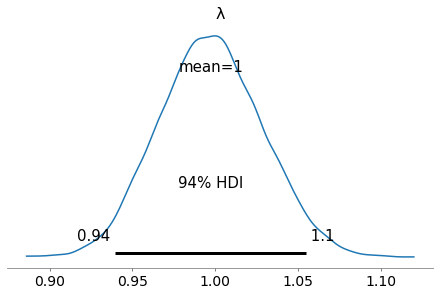The results are the same as before with the analytical posterior.

We should note that Bayesian estimators are ALWAYS biased due to their choice of prior, however, they can reduce the variance in our estimators. This is will become evident in the next example where we show another area where Bayesian method shine is when you have a limited amount of data, but a lot of domain knowledge.

Say we only have 20 sample points, we can calculate the MLE,

In :
mle(sample[-20:])

Out:
1.0526315789473684

Not too bad! However, the confidence interval is quite large,

In :
ci(sample[-20:])

Out:
(0.6101254949789889, 1.4951376629157478)

Let's define our model to be a Poisson-Exponential model where the prior distribution is an exponential distribution pictured below from PyMC3's site:It's unfortunate that these two distributions both use $\lambda$ for their paramaters, but I will do my best to make it clear which $\lambda$ I refer to. Using a larger $\lambda$ in the exponential prior gives us a smaller sample space while a smaller $\lambda$ in the exponential prior gives us a larger sampling space. Let's choose a define the prior $\exp(-1)$ and sample it using MCMC methods 500 times. We can then plot the posterior and the sampling space using the plot_trace method,

In :
with pm.Model() as model_2:
λ = pm.Exponential('λ', 1)
y = pm.Poisson('y', mu=λ, observed=list(sample[-20:-1]))
trace2 = pm.sample(500, tune=2000, cores=2, return_inferencedata=True)

az.plot_trace(trace2, var_names=['λ'])

Auto-assigning NUTS sampler...
Multiprocess sampling (2 chains in 2 jobs)
NUTS: [λ]

100.00% [5000/5000 00:11<00:00 Sampling 2 chains, 0 divergences]
Sampling 2 chains for 2_000 tune and 500 draw iterations (4_000 + 1_000 draws total) took 12 seconds.

Out:
array([[<AxesSubplot:title={'center':'λ'}>,
<AxesSubplot:title={'center':'λ'}>]], dtype=object)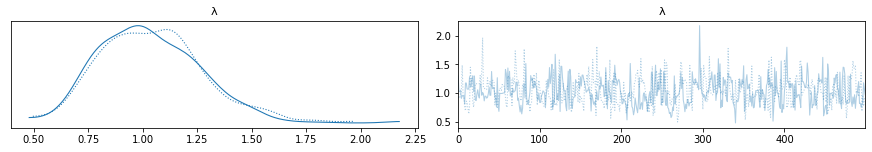We can then calculate the expected value of the posterior and the credible region,

In :
az.summary(trace2, kind="stats")

Out:
mean sd hdi_3% hdi_97%
λ 1.037 0.231 0.662 1.488

The values are nearly the same as MLE.

One thing to note is that we can see that the posterior isn't very well defined using only 500 MCMC steps. We can see that there seems to be some autocorrelation in the sample space.

In :
az.plot_autocorr(trace2)

Out:
array([<AxesSubplot:title={'center':'λ\n0'}>,
<AxesSubplot:title={'center':'λ\n1'}>], dtype=object)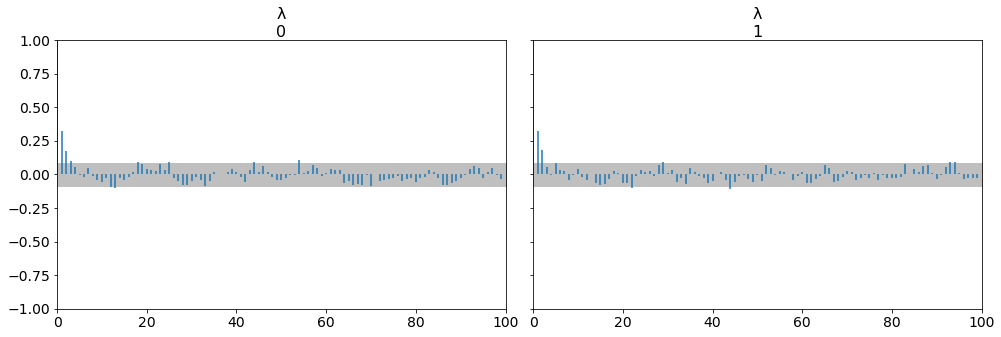Let's bump the number of samples up to 10,000 to see how the posterior distribution looks,

In :
with model_2:
trace3 = pm.sample(10000, tune=2000, cores=2, return_inferencedata=True)

az.plot_trace(trace3, var_names=['λ'])

Auto-assigning NUTS sampler...
Multiprocess sampling (2 chains in 2 jobs)
NUTS: [λ]

100.00% [24000/24000 00:31<00:00 Sampling 2 chains, 0 divergences]
Sampling 2 chains for 2_000 tune and 10_000 draw iterations (4_000 + 20_000 draws total) took 32 seconds.

Out:
array([[<AxesSubplot:title={'center':'λ'}>,
<AxesSubplot:title={'center':'λ'}>]], dtype=object)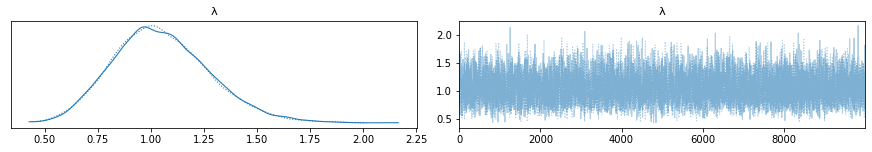We can see the posterior is pretty well defined, with a little skew right. Let's get the expected mean and credible interval,

In :
az.summary(trace3, kind="stats")

Out:
mean sd hdi_3% hdi_97%
λ 1.048 0.229 0.62 1.468

The Bayesian in method in this case isnt much better than the MLE, but credible interval is more narrow than the confidence interval.

We can also see the mode of the posterior distribution is nearly directly over 1.0, which is the correct value for our parameter.

Using the posterior mode as an estimator is called the Maximum A-Posteriori (MAP) and we can see the calculated value below,

In :
pm.find_MAP(model=model_2)

100.00% [6/6 00:00<00:00 logp = -26.451, ||grad|| = 6.1371]

Out:
{'λ_log__': array(-1.70517194e-07), 'λ': array(0.99999983)}

That's really good for only 20 data points!!

## 6. Connecting The Two Methods ¶

One way we can connect Bayesian methods with the MLE is by choosing a constant prior $C$ or uniform ($U(\theta)$ so long $\theta \, \geq \, \lambda$). Then MAP is the same as the MLE:

$$\max_{\lambda} \, P(\lambda \, \vert \, y_1, y_2, \ldots, y_n) \; = \; \frac{1}{m(y_1, y_2, \ldots, y_n)} \, \max_{\lambda} \, L(\lambda \, \vert \, y_1, y_2, \ldots, y_n)$$

We can show with PyMC3 by choosing the prior as a $U(10)$:

In :
with pm.Model() as model_3:
λ = pm.Uniform('λ', lower=0, upper=10)
y = pm.Poisson('y', mu=λ, observed=list(sample[-20:-1]))

pm.find_MAP(model=model_3)

100.00% [8/8 00:00<00:00 logp = -70.371, ||grad|| = 37.5]

Out:
{'λ_interval__': array(-2.14006616), 'λ': array(1.05263158)}

This the the same value as the MLE!

The Bernstein-von Miss Theorem shows rigorously that in the limit of large data Bayesian estimators and Maximum Likelihood estimators converge to the same thing.

## 7. Conclusions ¶

In this post I discussed frequentist and Bayesian estimation techniques applied to data from a Poisson distribution and covered how to quantity the uncertaintity in each method. I showed how to sample a probability distribution written in Scala from Python using Py4J. For frequentist methods I covered maximum likelihood estimation, its convergence and quantifying uncertaintity with confidence intervals using the Fisher information. I also covered using Bayesian estimators using PyMC3 and quantifying their uncertaintity with credible intervals using ArviZ. Finally we showed the connection between the maximum likelihood esimators and Bayesian estimators by choosing a flat prior

I leared a lot in creating this post and hope you enjoyed it!

In [ ]: